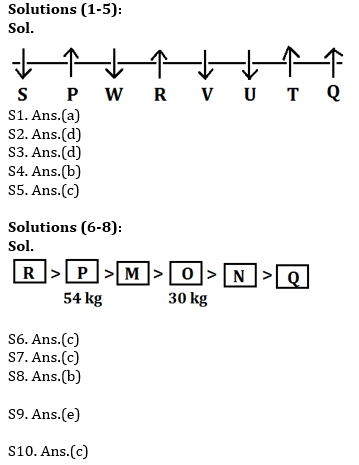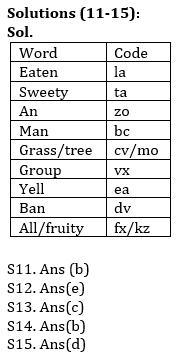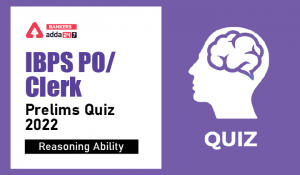Latest Banking jobs   »   Reasoning Ability Quiz For IBPS RRB...

# Reasoning Ability Quiz For IBPS RRB PO, Clerk Prelims 2021- 10th May

Directions (1-5): Study the following information carefully and answer the questions given below:
Eight persons are sitting in a row, in such a way some of them are facing south while some are facing north. V sits third to the left of Q. U is not an immediate neighbour of Q. P sits third to the right of V. W sits to the immediate right of P. W does not sits at any of the extreme end of the line. Only one person sits between R and U. P sits second to the left of R. S sits second to the right of W. Both the immediate neighbour of P faces the same direction to each other. Both the immediate neighbour of V faces the opposite direction to each other. S faces the same direction as W. T faces the same direction as R. V faces same direction as U. T faces north.

Q1. Who among the following sits immediate right of V?
(a) R
(b) T
(c) U
(d) S
(e) None of these

Q2. How many persons sit left of W?
(a) Two
(b) Four
(c) Three
(d) More than four
(e) None

Q3. Who among the following is an immediate neighbour of S?
(a) R
(b) W
(c) Q
(d) P
(e) None of these

Q4. Which of the following statement is true about Q?
(a) S is an immediate neighbor of Q
(b) W faces opposite direction to Q
(c) Only two persons are sitting between Q and R
(d) U sits third to the left of Q
(e) None of these

Q5. What is the position of T with respect to R?
(a)Second to the right
(b) Third to the left
(c) Third to the right
(d) Immediate left
(e) None of these

Directions (6-8): Read the given information carefully and answer the given questions.
Six boxes M, N, O, P, Q, and R are placed horizontally in a room, each box is of different weight. Weight of box R is a perfect cube of a number. Box M is heavier than O but lighter than R. Only one box is lighter than N. P is heavier than Q and its weight is 54 kg. O is not the lightest box. Weight of third lightest box is 30 kg. Not more than three boxes are lighter than M.

Q6. Which of the following box is lightest?
(a) Box O
(b) Box M
(c) Box Q
(d) Box R
(e) None of These

Q7. What can be the possible weight of box R?
(a) 27 Kg
(b) 8 Kg
(c) 64 Kg
(d) 55 kg
(e) None of these

Q8. Which of the following is the second heaviest box?
(a) Box O
(b) Box P
(c) Box M
(d) Box Q
(e) Box R

Q9. If in the number 683297514, all digits are arranged in increasing order from right to left than how many digits remain the same place in the new arrangement?
(a) Three
(b) Four
(c) none
(d) Two
(e) One

Q10. Which of the following elements should come in a place of ‘?’
MN4 OL6 QJ8 ?
(a) TG12
(b) SP12
(c) SH10
(d) LO12
(e) None of these

Directions (11-15): Study the information and answer the following questions:
In a certain code language
‘eaten sweety an man’ is written as ‘ la bc ta zo ’,
‘grass tree eaten group’ is written as ‘ cv vx la mo ’,
‘group an yell ban’ is written as ‘ zo dv ea vx’,
‘ban all sweety fruity’ is written as ‘fx ta kz dv ’,

Q11. What is the code for ‘man’ in the given code language?
(a) la
(b) bc
(c) ta
(d) zo
(e) None of these

Q12. What is the code for ‘tree’ in the given code language?
(a) cv
(b) vx
(c) la
(d) mo
(e) Cannot be determined

Q13. What is the code for ‘yell’ in the given code language?
(a) zo
(b) dv
(c) ea
(d) vx
(e) None of these

Q14. What is the code for ‘ban’ in the given code language?
(a) zo
(b) dv
(c) ea
(d) vx
(e) None of these

Q15. What would be the possible code for ‘grass fruity taste’ in the given code language?
(a) cv mo yu
(b) fx kz ua
(c) ua cv zo
(d) sn cv fx
(e) kz fx mo

Practice More Questions of Reasoning for Competitive Exams:

###### Study Plan for IBPS RRB PO/Clerk Prelims 2021

Solutions#### Congratulations!Incorrect details? Fill the form again here

•Reasoning Ability Quiz For IBPS Clerk/PO...
•Reasoning Ability Quiz For IBPS RRB PO C...
•Reasoning Ability Quiz For IBPS Clerk Pr...
•Reasoning Ability Quiz For IBPS RRB PO C...
•Reasoning Ability Quiz For IBPS RRB PO C...
•Reasoning Ability Quiz For IBPS Clerk Pr...